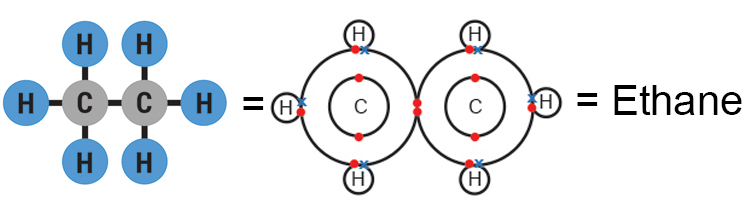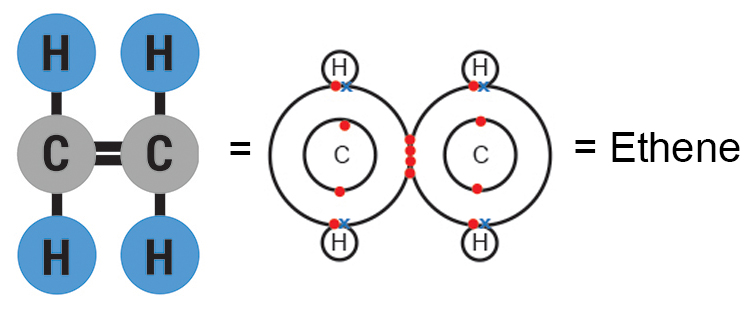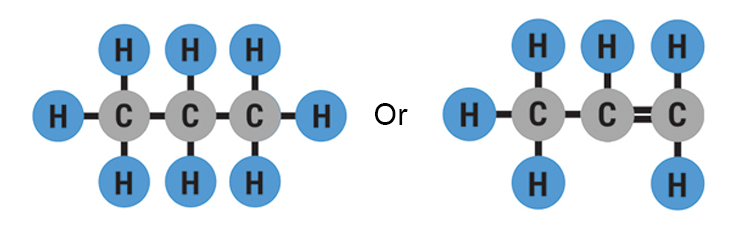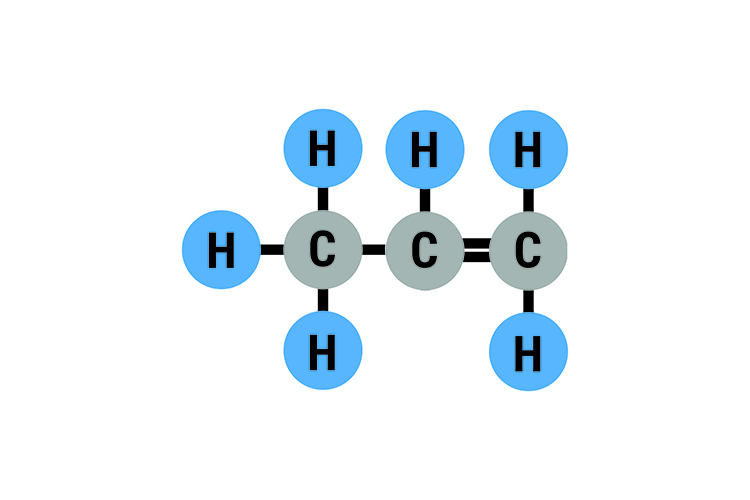# How to remember the hydrocarbons

Every carbon atom needs four connections to have a full outer shell.This can be achieved by adding hydrogen molecules as:Or you could join to a carbon molecule and hydrogen moleculesOr you could join to a carbon molecule with a double bond and hydrogen molecules.In an exam you need to be able to read a molecule diagram and know what they are.

For exampleC = Carbon atoms = 2

and 2 = ETH

 Also double bond = Alkene and Alkene = ENE

and you put the two together

ETH+ENE

ETHENE = C2H4

So the above molecule means ETHENE

Alternatively, you could be told you are dealing with Butane.

BUTANE

and you need to work out what the molecule looks like

BUT (BUTE) = 4 Carbon Atoms

ANE = ALKANE = Single Bond

and you put the two together

4 Carbon Atoms + Single Bond

Would look likeButane means C4H10

YOU NEED TO KNOW THAT

Meth   = 1 carbon atom

Eth       = 2 carbon atoms

Prop    = 3 carbon atoms

But      = 4 carbon atoms

and

-ane       = single carbon bond

-ene      = double carbon bond

-anol      = contains OH

Mammoth Chemistry

Links the numbers 1, 2, 3 and 4 to Meth, Eth, Prop and But by creating a picture of each number and linking that picture to Meth, Eth, Prop and But.

We learn that

1 = bun

2 = shoe

3 = tree

4 = door

(This is called the peg method).

and

 Meth = Me teeth Eth = Death Prop = Propeller But = Beautiful

We then connect the two

 1 = Bun Meth = Me teeth

Me teeth eating a bun (Meth = 1)

 2 = Shoe Eth = Death

Death wearing bright shoes (Eth = 2)

 3 = Tree Prop = Propeller

Propeller in a tree (Prop = 3)

 4 = Door But = Beautiful

Beautiful person in doorway (But = 4)

So now the word Prop means

Prop = Propeller = Tree = 3

Prop has 3 Carbon atoms

and this could mean we are dealing with eitherMammoth memory chemistry

Links the words single bond and double bond to the words "anes" and "enes" by creating a picture of "SINGLE" and "double bond" and linking that picture to "anes" and "enes".

We learn that

 Single = Shingle Anes (Alkanes) = Cane (Walking cane)

Walking canes standing in a shingle beach (single (bond) = anes)

we learn that

 Double bond = Two James Bonds enes (Alkenes) = Queen

Our two Queens need two James Bonds (Double Bond = ene)

So now the word Prop-ene means

Prop = Propeller = Tree = 3

(Prop has 3 Carbon atoms)

and

ene = Queen = Two James Bond = Double Bond

So from this we know we are dealing withThe following sections teach

The peg method

1  = bun

2  = shoe

3  = tree

4  = door

 Alkanes = Canes = Shingle = Single Alkenes = Queens = Two James Bonds = Double Bond Meth = Me teeth eating a bun = Meth is 1 Carbon Eth = Death with red shoes = Eth is 2 Prop = Propeller in a tree = Prop is 3 But = Beautiful in doorway = But is 4

So this allows you to know what is meant by methane, ethane, propane, and butane and also ethene, propene, and butene.